# Right angle resistor

New for March 2018!

You know that TV show, "Mythbusters?"  If you are an engineer, you probably think it is lame, as it was produced for the great unwashed masses.   Many episodes end in blowing something up, and the hosts saying "let's get it on" while trying to curve a bullet's path, when high school physics would tell you it is all a waste of time.

What about the myth that the corner square of a thin-film resistor counts as 50% of a normal square?  That's Microwaves101 Rule of Thumb #54.  Let's use some EM analysis to see how well this myth holds up.

## Example 1: Evaluation using layout editor

This analysis came from Hadrien.  He used Juspertor Layout Editor to create a resistor that has 1 ohm/square, then used the extraction tool to check the resistance.  Juspertor is practically free software, not some expensive EDA platform, so in Example 2 we will compare it to Axiem.

In order to set up a right-angle resistor for evaluation, you need at least three squares of resistor material, as shown in the desktop view below. The layout tool extracted 2.54 ohms (see lower right corner).  "Résistance" is French for "resistance", you might want to study the FFI in WWII to learn about the French résistance.  Allowing for the two straight sections at 1 ohm each, the corner section is 54% of the normal ohms-per-square of the material.  That's pretty close to the 1/2 value we used as a rule of thumb! In any case, if you were going to laser trim a resistor, you don't care if it comes out a little high...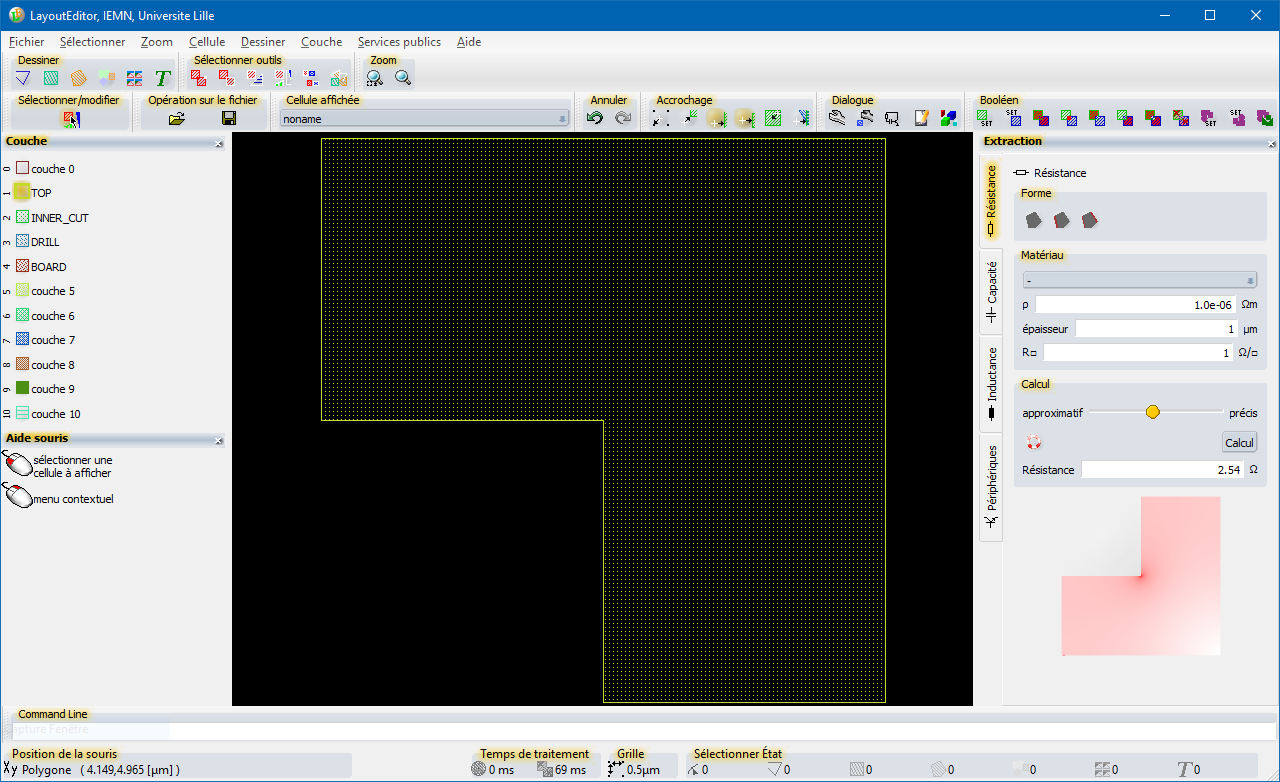## Example 2: Evaluation using 2.5D EM analysis

Here we will look at an example using Microwave Office Axiem 2.5D analysis. We opened a blank file and used the default layers of "thick metal" for conductor, and "tantalum" for resistor (nominally 50 ohms per square).  We changed the thick metal conductivity to "perfect" so that it does not affect the measurement.  The substrate is 100um GaAs, but that will not matter.  We constructed a two-port resistor, with three "squares" of 70 x 70um each.  We could have made a one-port circuit but that would have taken an extra minute to drop in a via and add nitride etch so that it connects to the thick metal traces.  Below is a 3D image of the overall circuit.  Although no overlap is really required between the resistor and conductor, it is good engineering practice to show it, otherwise someone might try to make a resistor without overlap and sue us when it comes out open circuited...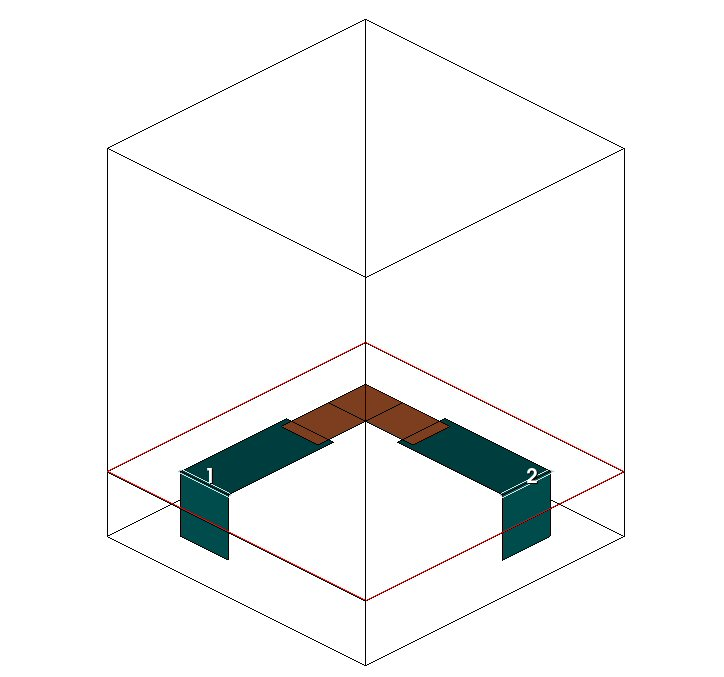Here is the first mesh we tried.  Note the overlap between resistor and thick metal; the perfect conductor will short out the resistor in these regions.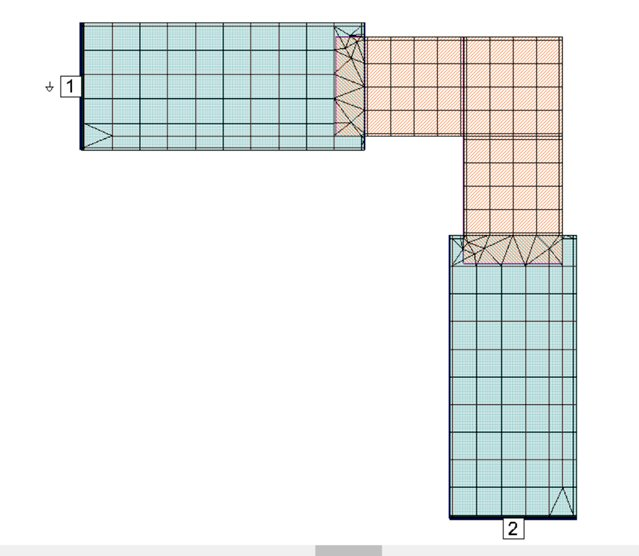The reflection coefficient is plotted on a Smith chart, plotted from 10 MHz to 100 MHz.  We can assume that 10 MHz is the "DC value" and that's where we placed the marker.  Keep in mind that Port 2 has a fifty ohm termination, and there are two straight sections to the resistor, and that in a fifty ohm Smith chart, "1" is equal to fifty ohms.  Subtracting 3 from the R value of 3.57, we get 57% of a square for the corner section in this case.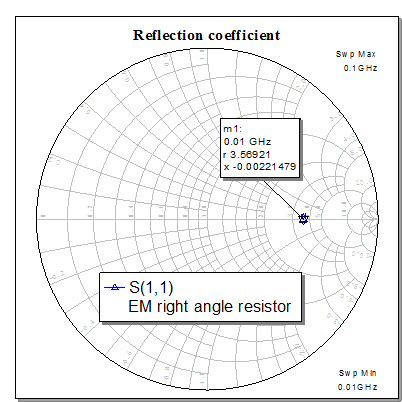Next we doubled the mesh.  If the resistance changes, tighter mesh should be closer to the correct answer. Note that there is no correct answer, everything we show on this page is an approximation to some degree or another.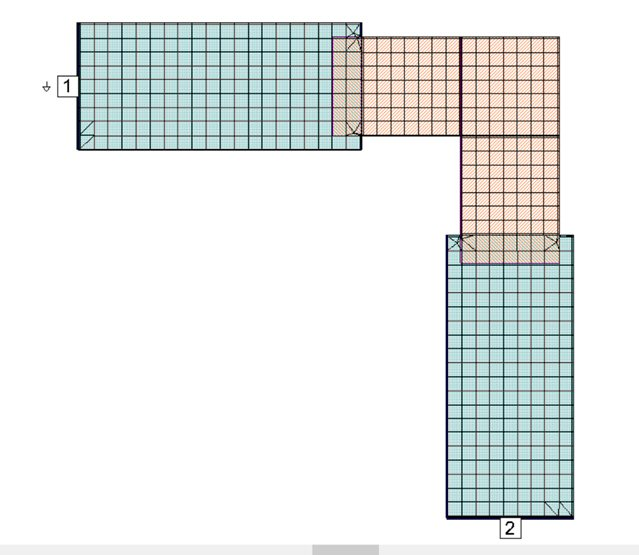With the second mesh, we see 56.4% of nominal for the corner section.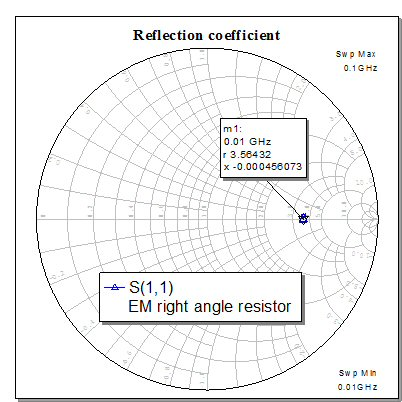Below, we doubled down on the meshing once more.  This took ten minutes to run, so we will stop here.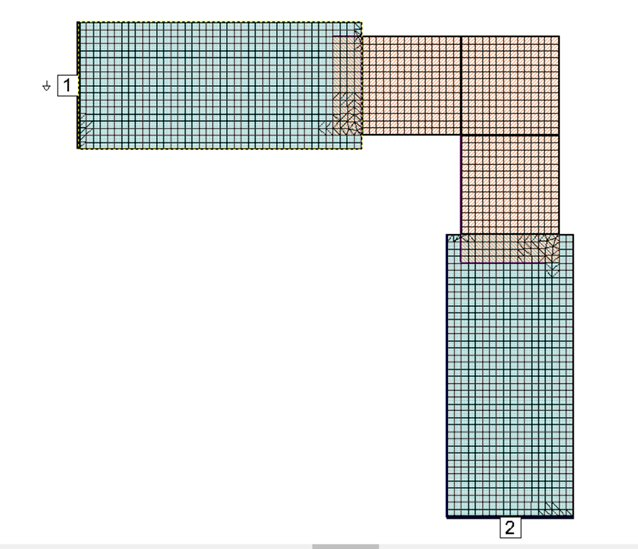With our final mesh, the corner resistor comes out at 56% of its value if it was fed staight through.  It seems like we are close to the asymptotic value, there was less change from Mesh 2 to Mesh 3 than there was from Mech 1 to Mesh 2.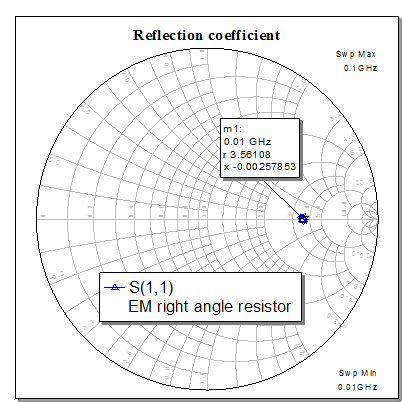Conclusions: the cheap layout editor came up with a value very close to the expensive EDA platform... there may actually be some difference caused by one simulation using 1 ohm per square, and the other using 50 ohm per square, maybe we should have run the exact same case.  In our estimation, we'll take the average of the two, and state that the value of a right-angle resistor is close to 56% of nominal, which is close to our original rule of thumb.  Which we just updated...

Author : Unknown Editor